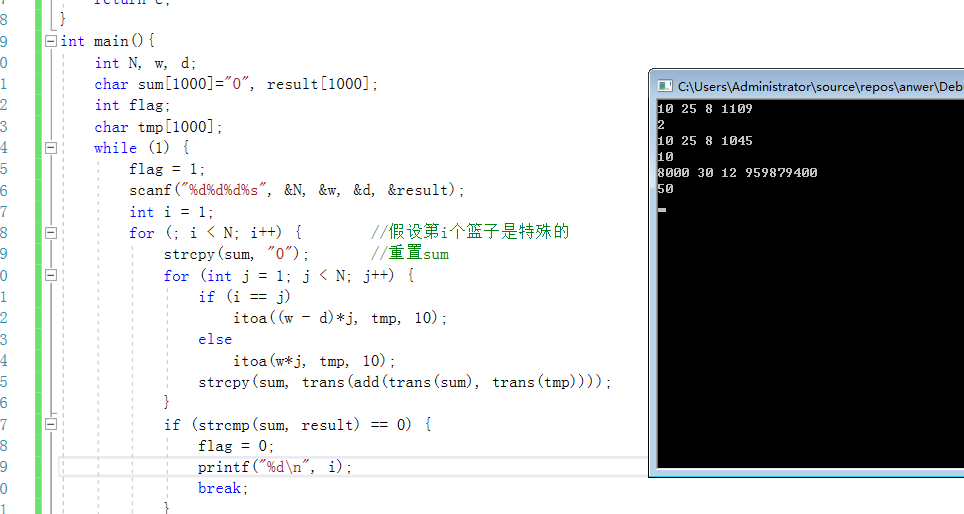2018-11-03 16:21

# 求教这个问题用C语言的实现

Problem Description
You are given N baskets of gold coins. The baskets are numbered from 1 to N. In all except one of the baskets, each gold coin weighs w grams. In the one exceptional basket, each gold coin weighs w-d grams. A wizard appears on the scene and takes 1 coin from Basket 1, 2 coins from Basket 2, and so on, up to and including N-1 coins from Basket N-1. He does not take any coins from Basket N. He weighs the selected coins and concludes which of the N baskets contains the lighter coins. Your mission is to emulate the wizard's computation.

Input
The input file will consist of one or more lines; each line will contain data for one instance of the problem. More specifically, each line will contain four positive integers, separated by one blank space. The first three integers are, respectively, the numbers N, w, and d, as described above. The fourth integer is the result of weighing the selected coins.

N will be at least 2 and not more than 8000. The value of w will be at most 30. The value of d will be less than w.

Output
For each instance of the problem, your program will produce one line of output, consisting of one positive integer: the number of the basket that contains lighter coins than the other baskets.

Sample Input
10 25 8 1109
10 25 8 1045
8000 30 12 959879400

Sample Output
2
10
50

• 写回答
• 好问题 提建议
• 关注问题
• 收藏
• 邀请回答

#### 2条回答默认 最新

•threenewbee 2018-11-21 12:10
已采纳
``````#include<stdio.h>
#include<string.h>
#define LL long long
int main()
{
int w,d,n;
int i;
LL m,sum,ans;
while(scanf("%d%d%d%lld",&n,&w,&d,&m)!=EOF)
{
sum=0;
for(i=1;i<n;i++)
sum+=i*w;
ans=sum-m;
if(ans==0)
printf("%d\n",n);
else
printf("%lld\n",ans/d);
}
return 0;
}
``````
已采纳该答案
评论
解决 无用
打赏 举报
•``````暴力破解，按顺序从1到N-1假设它为特殊篮子，如果最后求和如果跟输入的结果一样，说明假设成立，如果所有假设都不成立，那说明最后一个是特殊的篮子，另外，n<8000，需要用到大数加法，这里用我用char*，（用string操作比较简单）
如果有帮助请采纳=.=
``````
`````` #include<stdio.h>
#include<string.h>
#include<stdlib.h>
char* trans(char a[]) {         //字符串倒叙
char ch;
for (int i = 0; i < strlen(a)/2; i++) {
ch = a[i];
a[i] = a[strlen(a) - i - 1];
a[strlen(a) - i - 1] = ch;
}
return a;
}
char* add(char a[], char b[])   {       //把2个字符串相加
while (strlen(a) != strlen(b)) {
int al = strlen(a), bl = strlen(b);
if (al > bl)
b[bl] = '0';
else
a[al] = '0';
a[al+1] = b[bl+1] = '\0';
}
a[strlen(a)]=b[strlen(b)] = '\0';
int up = 0, sum;        //up储存进位
char c;
int i = 0;
for ( ; i < strlen(a); i++) {   //使用字符串模拟大数加法
sum = a[i] - '0' + b[i] - '0' + up;
up = sum / 10;
sum %= 10;
c[i] = char(sum+'0');
c[i + 1] = '\0';
}
if (up > 0) {
c[i] = up + '0';
c[i + 1] = '\0';
}
return c;
}
int main(){
int N, w, d;
char sum="0", result;
int flag;                       //用于记录是否有假设成立
char tmp;
while (1) {
flag = 1;
scanf("%d%d%d%s", &N, &w, &d, &result);
int i = 1;
for (; i < N; i++) {        //假设第i个篮子是特殊的
strcpy(sum, "0");       //重置sum
for (int j = 1; j < N; j++) {
if (i == j)
itoa((w - d)*j, tmp, 10);
else
itoa(w*j, tmp, 10);
}
if (strcmp(sum, result) == 0) {
flag = 0;
printf("%d\n", i);
break;
}
}
if (flag)
printf("%d\n", N);
}
return 0;
}
``````评论
解决 无用
打赏 举报